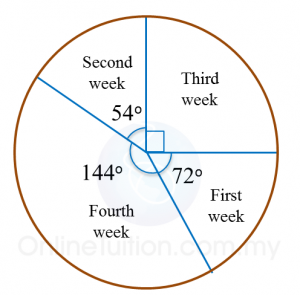# 12.2.4 Data Handling, PT3 Focus Practice

Question 7:
Table below shows the number of companies that gave contributions to an orphan fund over a period of five days.

 Days Monday Tuesday Wednesday Thursday Friday Number of companies 8 14 m 17 11

It is given that 20% of the total contribution was made on Tuesday.
Calculate the value of m

Solution
:
Let C = Total contribution
$\begin{array}{l}\frac{20}{100}×C=14\\ \text{}C=14×\frac{100}{20}\\ \text{}=70\\ m=70-\left(8+14+17+11\right)\\ \text{}=70-50\\ \text{}=20\end{array}$

 
 
   
Question 8:
 
Diagram below shows an incomplete line graph of the number of eggs sold in four weeks. The number of eggs sold on week 1 is 2000 and 4000 on week 4.(a) Complete the line graph in the Diagram.
 (b) Complete the pie chart in the second Diagram to represent sales from Week 1 to Week 4.Solution:
 (a)(b)